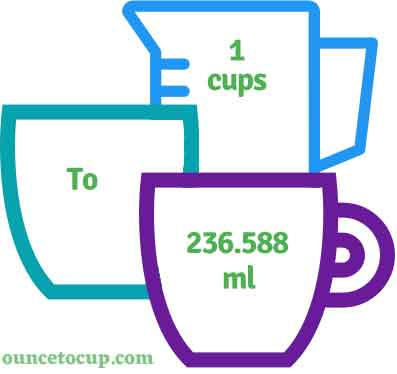# Cups to Milliliters (cup to ml conversion)

Are you cooking your favorite dish? The detailed chart in the recipe includes the calculation of 1 cups to ml conversion? Do not worry; check this conversion tool to find how many 1 cups equal to ml in a minute. This 1 cup to ml converter gives an exact measurement for any recipe you prepare.

Cup Value:

Cups

Milliliter Value:

ml

1 Cup = 236.588 Milliliter
(1 c = 236.588 ml)

Try our auto 1 cup to milliliter calculator (Without Convert Button), Just change the first field value and you got final value.## How many ml is a cup?

We know that the volume value of 1 c is equal to 236.588 ml. If you want to convert 1 cup to an equal number of ml, just multiply the volume value by 236.588. Hence, 1 Cup is equal to 236.588 ml.

The Answer is: 1 US Cups = 236.588 US ml

1 c = 236.588 ml

Many of them try to search or find an answer for what is 1 cups in ml? So, we’ll start with 1 c to ml conversion to know how big is 1 c.

## How To Calculate 1 cup to ml?

To calculate 1 cups to an equal number of milliliter, simply follow the steps below.

Fluid Cups to Milliliters formula is:

Milliliter = Fluid Cup * 236.588

Assume that we are finding out how many ml were found in 1 fl c of water, multiply by 236.588 to get the result.

Applying to Formula: ml = 1 c * 236.588 = 236.588 ml.

## How To Convert c to ml?

• To convert fluid cups to ml,
• Simply multiply the us cup value by 236.588.
• Applying to the formula, ml = 1 cups * 236.588 [1x236.588].
• Hence, 1 cups is equal to 236.588 ml.

## Some quick table references for cups to milliliter conversions:

Cup [c]Milliliter [ml]
1 cup236.588 ml
2 cup473.176 ml
3 cup709.764 ml
4 cup946.352 ml
5 cup1182.94 ml
6 cup1419.528 ml
7 cup1656.116 ml
8 cup1892.704 ml
9 cup2129.292 ml
10 cup2365.88 ml
11 cup2602.468 ml
12 cup2839.056 ml
13 cup3075.644 ml
14 cup3312.232 ml
15 cup3548.82 ml

## Reverse Calculation: How many cups are in a ml?

• To convert 1 ml to c,
• Simply divide the 1 ml by 236.6.
• Then, applying the formula, cup = 1 ml / 236.6 [1/236.6 = 0.00422675].
• Hence, 1 ml is equal to 0.00422675 c.

### Related Converter:

Formula: Cup to ml

ml = cup * 236.588

Applying to Formula,

ml = 1*236.588 = 236.588

1 c = 236.588 ml